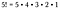# Combinations vs Permutations

We throw around the term “combination loosely, and usually in the wrong way. We say things like, “Hey, what’s your locker combination?” but what we really ought to be sayings is “Hey, what’s your locker permutation?”

So what’s the difference? And what exactly is a permutation? Watch or read on :)

# How To Tell the Difference

The difference between combinations and permutations is ordering. With permutations we care about the order of the elements, whereas with combinations we don’t.

For example, say your locker “combo” is 5432. If you enter 4325 into your locker it won’t open because it is a different ordering (aka permutation).

The permutations of 2, 3, 4, 5 are:

Your locker “combo” is a specific permutation of 2, 3, 4 and 5. If your locker worked truly by combination, you could enter any of the above permutations and it would open!

# Calculating Permutations with Ease

Suppose you want to know how many permutations exist of the numbers 2, 3, 4, 5 without listing them like I did above. How would you accomplish this?

Let’s use a line diagram to help us visualize the problem.

We want to find how many possible 4-digit permutations can be made from four distinct numbers. Begin by drawing four lines to represent the 4 digits.

The first digit can be any of the 4 numbers, so place a “4” in the first blank.

Now there are 3 options left for the second blank because you’ve already used one of the numbers in the first blank. Place a “3” in the next space.

For the third position, you have two numbers left.

And there is one number left for the last position, so place a “1” there.

## The Multiplication Principle

Using the Multiplication Principle of combinatorics, we know that if there are x ways of doing one thing and y ways of doing another, then the total number of ways of doing both things is x•y. That means we need to multiply to find the total permutations.

This is a great opportunity to use shorthand factorial notation (!):

There are 24 permutations, which matches the listing we made at the beginning of this post.

# Permutations with Repetition

What if I wanted to find the total number of permutations involving the numbers 2, 3, 4, and 5 but want to include orderings such as 5555 or 2234 where not all of the numbers are used, and some are used more than once?

How many of these permutations exist?

This turns out to be a simple calculation. Again we are composing a 4-digit number, so draw 4 lines to represent the digits.

In the first position we have 4 number options, so like before place a “4” in the first blank. Since we are allowed to reuse numbers, we now have 4 number options available for the second digit, third digit, and fourth digit as well.

That’s the same as:

By allowing numbers to be repeated, we end up with 256 permutations!

# Choosing a Subset

Let’s up the ante with a more challenging problem:

How many different 5-card hands can be made from a standard deck of cards?

In this problem the order is irrelevant since it doesn’t matter what order we select the cards.

We’ll begin with five lines to represent our 5-card hand.

Assuming no one else is drawing cards from the deck, there are 52 cards available on the first draw, so place “52” in the first blank.

Once you choose a card, there will be one less card available on the next draw. So the second blank will have 51 options. The next draw will have two less cards in the deck, so there are now 50 options, and so on.

That’s 311,875,200 permutations.

That’s permutations, not combinations. To fix this we need to divide by the number of hands that are different permutations but the same combination.

This is the same as saying how many different ways can I arrange 5 cards?Note: This is mathematically similar to finding the different permutations of our locker combo

So the number of five-card hands combinations is:

# Rewriting with Factorials

With a little ingenuity we can rewrite the above calculation using factorials.

We know 52! = 52•51•50•…•3•2•1, but we only need the products of the integers from 52 to 48. How can we isolate just those integers?

We’d like to divide out all the integers except those from 48 to 52. To do this divide by 47! since it’s the product of the integers from 47 to 1.

Make sure to divide by 5! to get rid of the extra permutations:

There we go!

Now here’s the cool part → we have actually derived the formula for combinations.

# Combinations Formula

If we have n objects and we want to choose k of them, we can find the total number of combinations by using the following formula:

For example, we have 52 cards (n=52) and want to know how many 5-card hands (k=5) we can make.

Plugging in the values we get:

Which is exactly what we found above!

Often times you’ll see this formula written in parenthesis notation, like above, but some books write it with a giant C:

# Permutations Formula

The formula for permutations is similar to the combinations formula, except we needn’t divide out the permutations, so we can remove k! from the denominator:

Alright, there you go! A quick run-down of the basics of combinatorics. Hope this helps you out :)

## ❤ STAY CONNECTED ❤

Stay up-to-date with everything Math Hacks is up to!

## Math Hacks

Tutorials with a fresh perspective.

Written by

## Brett Berry

Check out my YouTube channel “Math Hacks” for hands-on math tutorials and lots of math love ♥️## Math Hacks

Tutorials with a fresh perspective.